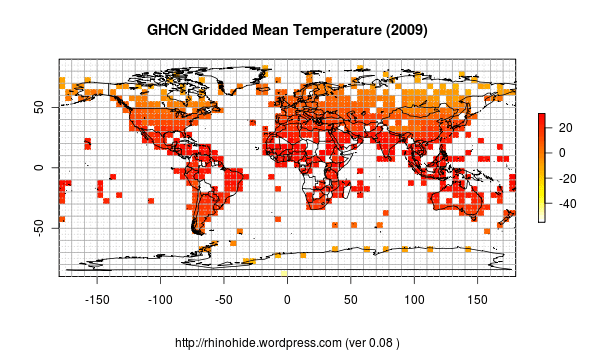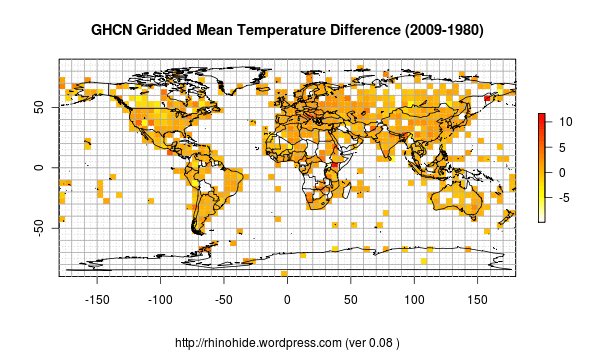Home > GHCN, trb > trb-0.08: Pretty Pictures

## trb-0.08: Pretty Pictures

2010 July 24

Introduction

There’s a green one and a pink one
And a blue one and a yellow one,
And they’re all made out of ticky tacky
And they all look just the same.

Mosherology

Mosher pointed out the ‘raster’ package some days ago when I asked about plotting grids. It has the right stuff to make pretty pictures.

However, I haven’t seen a way to have irregularly spaced latitudinal zones. The raster expects a regular array of latitudes and longitudes, so I had to change the zonal portion of the code back to using a common step size. So to account for that, I have added a matrix of weights based on the fractional area of the cell grid (box). This zonal weighting is not used for the pretty pictures, however, which come straight from the raster grid which is defined by the tapply function.

The ‘raster’ package required I install the Ubuntu packages “libgdal1-dev” and “proj.” I’m actually kind of excited by the raster/rgdal/sp and related packages. It looks very likely that I will be able to put up some very straightforward (and cleaner) code to do the meta-data lookups that I have previously been doing with my modified and extended Java netcdf classes.

Code

```###############################################################################
# trb 0.08 : 2010 07 23 : Raster / Pretty Pictures / Fractional Area Weights
# trb 0.07 : 2010 07 18 : Performance Refactor (22 seconds with data read)
# trb 0.06 : 2010 07 17 : Performance Refactor (3x faster, still 8hrs for all)
# trb 0.05 : 2010 07 14 : Gridding by Latitude and Longitude
# trb 0.04 : 2010 07 13 : Proportional Latitudinal Bands
# trb 0.03 : 2010 07 12 : Weighted Latitudinal Bands
# trb 0.02 : 2010 07 11 : Simple Latitudinal Bands
# trb 0.01 : 2010 07 10 : GHCN Simple Mean Average
# https://rhinohide.wordpress.com
###############################################################################
ver <- "0.08"
read <- FALSE # set to FALSE if this is your first time through
#read <- TRUE # set to TRUE if you have previous data to read

flag_latarea <- TRUE # set to TRUE if you want equal area lat bands
flag_latarea <- FALSE # set to FALSE if you want equal step lat bands

###############################################################################
# Read Data Files
###############################################################################
# ftp://ftp.ncdc.noaa.gov/pub/data/ghcn/v2/v2.mean
# ftp://ftp.ncdc.noaa.gov/pub/data/ghcn/v2/v2.temperature.inv
###############################################################################

# this flag is to indicate if we must read for the first time
# or if we can read the data from previous runs

#------------------------------------------------------------------------------
# Temperature File (GHCN formatted monthly means)
#------------------------------------------------------------------------------

# mm for 'monthly means'
mmwids=c(11,1,4,5,5,5,5,5,5,5,5,5,5,5,5)
mmcols=c("id","dupe","year","jan","feb","mar","apr","may","jun",
"jul","aug","sep","oct","nov","dec")

# read the file
mm <- read.fwf("../data/v2.mean", widths=mmwids, col.names=mmcols,

#------------------------------------------------------------------------------
# Inventory File
#------------------------------------------------------------------------------

# there are five occurrances of the pound hash in v2.temperature.inv
# R scripts chokes on reading those, remove them first

invcols <- c("id","name","lat","lon","alt","elev","ru","pop","terr","arid",
invwids <- c(11,32,6,8,5,5,1,5,2,2,2,2,1,2,16,1,5)

###############################################################################
# Set up arrays for latitudinal banding
###############################################################################

hindex=new.env(hash=TRUE)
hlat=new.env(hash=TRUE)
hlon=new.env(hash=TRUE)
for (i in 1:nrow(inv)) {
hindex[[as.character(inv[i,1])]] <- i
hlat[[as.character(inv[i,1])]] <- inv[i,3]
hlon[[as.character(inv[i,1])]] <- inv[i,4]
}

mmlat <- rep(NA,nrow(mm))
mmlon <- rep(NA,nrow(mm))

for (i in 1:nrow(mm)) {
mmlat[i] <- hlat[[as.character(mm[i,1])]]
mmlon[i] <- hlon[[as.character(mm[i,1])]]
}

# add inv lat lon
mm1 <- cbind(mm,mmlat,mmlon)

# general formula for a set number of latitude bands of equal area
nbands <- 36
bands <- rep(NA,nbands)
b = 90                      # start with santa
bands=b
bands[nbands+1]=-90         # rounding errors at nbands ~ 32
for (i in 1:(nbands-1)) {
if (flag_latarea) {
# equal area lat bands
a = asin(sin(b*pi/180)-2/nbands)
b = a * 180 / pi
bands[i+1] = b
} else {
# equal lat step size
bands[i+1] = 90 - (180/nbands) * i
}
}
bands

# 'bars' are the longitudinal edges of the grid
# calculate the bars long now so we can just lookup later

nbars <- nbands * 2        # this could be set to any number
bars <- matrix(NA,nbands+1,nbars+1)
bars[,1] = -180            # start in Howland Island and move east ;-)
for (i in 1:(nbands+1)) {
for (j in 1:nbars) {
bars[i,j+1] = 360*(j/nbars)-180
}
}

# weights for each cell
weights <- matrix(NA,nbands,nbars)
for (i in 1:(nbands)) {
for (j in 1:nbars) {
# assumes equal cells within a lat band
weights[i,j]  <- abs((sin(bands[i]*pi/180) - sin(bands[i+1]*pi/180)))/(2*nbars)
}
}
sum(weights[,]) # should equal 1

#------------------------------------------------------------------------------
# assign each record to a grid point
#------------------------------------------------------------------------------
mmlat <- rep(NA,nrow(mm1))
mmlon <- rep(NA,nrow(mm1)) for (i in 1:nrow(mm1)) {     mmlat[i] = sum(bands>mm1[i,16])
mmlon[i] = sum(bars[mmlat[i],]<- cbind(mmlat,mmlon,mm1[,3],rowMeans(mm1[,4:15],na.rm=T)/10)
colnames(mm2) <- c("ilat","ilon","yr","mean")

#------------------------------------------------------------------------------
# set up the range of years to calculate
#------------------------------------------------------------------------------
#year0 <- min(mm1[,3])
year0 <- 1880              # 1880 is an arbitrary start date
#year0 <- 2008              # use a short range during developement
year1 <- max(mm1[,3])
range <- year1 - year0 + 1

#------------------------------------------------------------------------------
# trim the matrix to the span of years
#------------------------------------------------------------------------------
idx <- mm2[,3]>= year0 & mm2[,3]<=year1
mm2 <- mm2[idx,]
mm2[1,]

#------------------------------------------------------------------------------
# clean up
#------------------------------------------------------------------------------
mm <- NULL
mm1 <- NULL
mmlat <- NULL
mmlon <- NULL
inv <- NULL
hlat <- NULL
hlon <- NULL

#------------------------------------------------------------------------------
# write the data
#------------------------------------------------------------------------------

write.table(mm2,".Rmm2",row.names=F,col.names=T)
write.table(bands,".Rbands",row.names=F,col.names=F)
write.table(bars,".Rbars",row.names=F,col.names=F)
write.table(weights,".Rweights",row.names=F,col.names=F)
write.table(c(year0,year1),".Ryears",row.names=F,col.names=F)

} else {

#------------------------------------------------------------------------------
# read the data
#------------------------------------------------------------------------------

nbands <- length(bands) -1
nbars <- ncol(bars) -1
year0 <- yrs
year1 <- yrs
range <- year1 - year0 + 1

} # end if for data

# this is a trick to ensure that some value (NA) exists for every lat band
# required to ensure that the tapply mean factors every lat band
a1 <- matrix(NA,nbands,4)
a1[,1] <- rep(1:nbands)
a1[,2] <- 1
a1[,3] <- year0
colnames(a1) <- c("ilat", "ilon", "yr", "mean")
mm2 <- rbind(mm2,a1)

###############################################################################
# Calculate Annual Mean Average
###############################################################################

#------------------------------------------------------------------------------
# initialize some vectors to hold annual results
#------------------------------------------------------------------------------
lyear <- rep(NA, range)
lmean <- matrix(NA,nbands,range)
lcount <- matrix(NA,nbands,range)
gmean <- array(NA, dim=c(nbands,nbars,range))
gcount <- array(NA, dim=c(nbands,nbars,range))

gmean <- tapply(mm2[,4], list(mm2[,1],mm2[,2],mm2[,3]), mean, na.rm=T)
lyear=seq(year0:year1)

#------------------------------------------------------------------------------
# loop through the range of years
#------------------------------------------------------------------------------
for (y in 1:range) {    # loop through each year
yr <- year0  + y - 1
for (i in 1:nbands) {    # loop through each lat band
lmean[i,y] = mean( gmean[i,,y], na.rm=T )
}

# use a mask to adjust for empty zones
# if only 75% of zones have data, divide by 75%
mask <- ! is.na (lmean[,y])

# the next two are equilvalent
# ameans[y]= sum(lmean[,y],na.rm=T) / (sum(mask) * sum(weights[mask,]))
ameans[y]= mean(lmean[,y],na.rm=T) / sum(weights[mask,])

lyear[y]=yr    # keep track of the ranges of years
}

#------------------------------------------------------------------------------
# calculate the global land temperature
#------------------------------------------------------------------------------

# since each band is weighted,
ameans=colMeans(lmean,na.rm=T)
mean(ameans, na.rm=T) # 10.94579

###############################################################################
# Raster
###############################################################################

library(sp)
library(rgdal)
library(raster)
library(maps)
r <- raster(nrows=nbands,ncols=nbars,
xmn=bars[1,1],xmx=bars[1,nbars+1],
ymn=bands[nbands+1],ymx=bands,
crs="+proj=longlat +datum=WGS84")
# set a year to display
y <-130
r <- setValues(r,as.vector(t(gmean[,,y])),layer=1)

img=paste("trb-",ver,"-grid-",lyear[y],".png", sep="")
tit=paste("GHCN Gridded Mean Temperature (",lyear[y],")",sep="")
subt=paste("https://rhinohide.wordpress.com (ver ",ver," )",sep="")
png(img)

plot(r,col=rev(heat.colors(100)), main=tit, sub=subt)
grid(nx=nbars,ny=nbands,col="lightgray")
grid(nx=nbars/2,ny=nbands/2,col="darkgray",lty="solid")

# display a diff of two years
a1 <-101
a2 <-130
r <- setValues(r,as.vector(t(gmean[,,a2]-gmean[,,a1])),layer=1)

img=paste("trb-",ver,"-grid-",lyear[a2],"-",lyear[a1],".png", sep="")
tit=paste("GHCN Gridded Mean Temperature Difference (",lyear[a2],"-",lyear[a1],")",sep="")
subt=paste("https://rhinohide.wordpress.com (ver ",ver," )",sep="")
png(img)

plot(r,col=rev(heat.colors(100)), main=tit, sub=subt)
grid(nx=nbars,ny=nbands,col="lightgray")
grid(nx=nbars/2,ny=nbands/2,col="darkgray",lty="solid")

quit()```

trb-0.08.R

Pretty Pictures

I realize the color scheme isn’t all that it could be. I’m still fiddling with it.The next one is a difference image: taking the ‘temps’ from 2009 and subtracting the temps in 1980. Unfortunately, this doesn’t show a trend between these two years, just the difference in endpoints.1. 2010 July 24 at 10:17 am

I like Nick Stokes’ pretty picture today better.

Don’t you have more colorbar to use? The resolution of this one is pretty bad.

I wonder if there are any interesting side questions to poke at, while you’re still using absolutes and not anomalies.

2. 2010 July 25 at 7:13 am

I had to take Nick out of the ‘infrequent’ list of bloggers yesterday.

Still haven’t learned how to make my own colorbar or to manually set the scale, but I did find that ‘rainbow’ makes a better display.

I can probably retain the ability to do both abs T or anomalies.

3. 2010 July 26 at 3:41 pm

I’m gunna have to catch up on the graphics side of things ! I dont have the legends and color thing down. hehe.

Glad you liked raster.. The rgdal stuff is cool as well so I’m looking forward to playing with that.

WRT irregularly spaced latitudes. You could make a raster stack, I think stacks allow differently framed

WRT the area weights. If you have a raster, then you can automatically get the area for
each grid Areas<-area(object)

You can also use the raster functions assign a station to a grid cell.

weights need to be calculated on a per month basis, at least that is how Zeke and I did it ( I copied him).. Not sure if your code is doing that.

4. 2010 July 26 at 8:14 pm

Steven: WRT irregularly spaced latitudes. You could make a raster stack, I think stacks allow differently framed

That’s a pretty good idea! One layer per lat zone.

Yeah, caught the area function reading docs yesterday.

The value of assigning a station to a grid cell isn’t clear to me yet.

Area weights need to be calculated per month? Makes no sense to me. Does the geometry of the earth change?

5. 2010 August 21 at 8:52 pm

On assigning stations to grids:

You take the stations (coords) and do getCellXY; that tells to the CELL number.

You then Average PER CELL. let raster do your binning

Area averaging

In a given month say you have 10 cells reporting. The total area of those 10 is 1Million
the weight of a given cell is cellarea/million

Next month you have 100 cells reporting: total area is say 5 million
the weight of a given cell is then cell area/5 million.

So each month you have to figure out the total AREA reported. and then the fration of area
each cell is.

if 1 cell reported, then the cell would have a 100% weight for that month

1. No trackbacks yet.